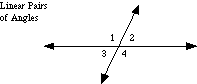index: click on a letter A B C D E F G H I J K L M N O P Q R S T U V W X Y Z A to Z index index: subject areas numbers & symbols sets, logic, proofs geometry algebra trigonometry advanced algebra & pre-calculus calculus advanced topics probability & statistics real world applications multimedia entrieswww.mathwords.com about mathwords website feedback

 Linear Pair of Angles A pair of adjacent angles formed by intersecting lines. Angles 1 and 2 below are a linear pair. So are angles 2 and 4, angles 3 and 4, and angles 1 and 3. Linear pairs of angles are supplementary.